Courses

# Important Formulae: Interests CAT Notes | EduRev

## Quant : Important Formulae: Interests CAT Notes | EduRev

The document Important Formulae: Interests CAT Notes | EduRev is a part of the Quant Course Quantitative Aptitude (Quant).
All you need of Quant at this link: Quant

• Amount = Principal + Interest
• Simple Interest = PNR/100
• Compound Interest =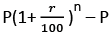• Population formula P’ =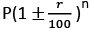• Depreciation formula = Initial Value x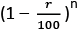EduRev's Tip: SI and CI are same for a certain sum of money (P) at a certain rate (r) per annum for the first year. The difference after a period of two years is given by
⇒ Δ = PR2/1002

Growth and Growth Rates
Absolute Growth = Final Value – Initial Value
Growth rate for one year period  =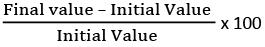SAGR or AAGR =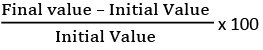CAGR =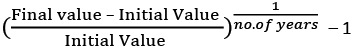EduRev's Tip: If the time period is more than a year, CAGR < AAGR. This can be used for approximating the value of CAGR instead of calculating it.

Offer running on EduRev: Apply code STAYHOME200 to get INR 200 off on our premium plan EduRev Infinity!

## Quantitative Aptitude (Quant)

116 videos|131 docs|131 tests

,

,

,

,

,

,

,

,

,

,

,

,

,

,

,

,

,

,

,

,

,

;